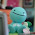## Sunday, January 16, 2011

### Karen's Final Percent Post

What is a Percent?
•number out of a hundred (50% means 50 out of a hundred, 50/100 or 0.50)

4.1 Representing Percents:
To represent a percent you can use 100 grids. One shaded grid that has all the squares shaded in represents 100%.
To show a percent that is larger then 100% you shade in more then on grid.
To represent a fractional percent between 0% and 1% you shade in a part of one square.
To represent a fractional percent greater than 1% shade squares from a hundred grid to show the whole number and part of on square from the grid to show the fraction.

4.2 Fractions, Decimals, Percents:
Fractions, decimals and percents can be used to represent numbers in various situations.
Percents can be written as fractions and decimals.
Example:
1/2% = 0.5% 150% = 150/100 42 3/4% = 42.75%
0.5 = 0.5/100 = .005 1.5 or 1 1/2 42.75% = 42.75/100 = 0.4275

4.3 Percent of a Number:
You can use some mental math stratagies such as doubling, halving, doubling or dividing by ten to find percents of some numbers.
To calculate the percent if a number write the percent as a decimal and then multiply by the number.
Example: 12 1/2% f 50 = 0.125 x 50 = 6.25 ( 12 1/2% = 12.5% = 0.125

4.4 Combining Percents:
Percents can be combined by adding to slove problems (eg: 5% + 7% = 12%).
To calculate the increase of a number:
-You can add the combined percent amount to the original number (eg: 12% of 100 = 0.12 x 100 = 12).
-You can multiply the original number by a single percent greater than 100 (eg: 112% of 100 = 1.12 x 100 = 112).
Percents of percents can be used to determine amounts that result from consecutive percent increases or decreases.

1.